# Stoich Flashcards

Set Details Share
created 10 years ago by hdiwza
3,283 views
updated 10 years ago by hdiwza
Page to share:
Embed this setcancel
COPY
code changes based on your size selection
Size:
X

1

What amount of oxygen, O2, (in moles) contains 1.8×10^22 molecules?
A. 0.0030
B. 0.030
C. 0.30
D. 3.0

B

2

Which compound has the empirical formula with the greatest mass?
A. C2H6
B. C4H10
C. C5H10
D. C6H6

B

3

__C2H2(g) + __O2(g) → __ CO2(g) + __ H2O(g)
When the equation above is balanced, what is the coefficient for oxygen?
A. 2
B. 3
C. 4
D. 5

D

4

3.0 dm3 of sulfur dioxide is reacted with 2.0 dm3 of oxygen according to the equation below. 2SO2(g) + O2(g) → 2SO3(g)
What volume of sulfur trioxide (in dm3) is formed? (Assume the reaction goes to completion and all gases are measured at the same temperature and pressure.)
A. 5.0
B. 4.0
C. 3.0
D. 2.0

C

5

What will happen to the volume of a fixed mass of gas when its pressure and temperature (in Kelvin) are both doubled?
A. It will not change.
B. It will increase.
C. It will decrease.
D. The change cannot be predicted.

A

6

What volume (in dm3) of 0.30 mol dm–3 NaCl solution can be prepared from 0.060 mol of solute?
A. 0.018
B. 0.2
C. 0.50
D. 5.0

B

7

Ag(s) + NO3–(aq) + H+(aq)  Ag+(aq) + NO(g) + H2O(l)
When the oxidation-reduction equation above is balanced, what is the coefficient for H+(aq)?
A.1
B. 2
C.3
D.4

D

8

What amount (in moles) is present in 2.0 g of sodium hydroxide, NaOH?
A. 0.050
B. 0.10
C. 20
D. 80

A

9

A hydrocarbon contains 90% by mass of carbon. What is its empirical formula?
A. CH2
B. C3H4
C. C7H10
D. C9H10

B

10

Copper can react with nitric acid as follows.
3Cu +_HNO3  _Cu(NO3)2 +_H2O + _NO
What is the coefficient for HNO3 when the equation is balanced?
A. 4
B. 6
C. 8
D. 10

C

11

Lithium hydroxide reacts with carbon dioxide as follows. 2LiOH + CO2 → Li2 CO3 + H2O
What mass (in grams) of lithium hydroxide is needed to react with 11 g of carbon dioxide?
A. 6
B. 12
C. 24
D. 48

B

12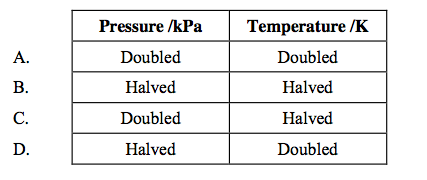Which change in conditions would increase the volume of a fixed mass of gas?

D

13

Which solution contains the smallest amount of H+ ions?
A. 10.0 cm3 of 0.250 mol dm–3 HCl
B. 20.0 cm3 of 0.250 mol dm–3 HCl
C. 10.0 cm3 of 0.500 mol dm–3 HCl
D. 10.0 cm3 of 0.250 mol dm–3 H2SO4

A

14

How many hydrogen atoms are contained in one mole of ethanol, C2H5OH?
A. 5
B. 6
C. 1.0×10^23
D. 3.6×10^24

D

15

The percentage by mass of the elements in a compound is
C = 72%, H = 12%, O = 16%.
What is the mole ratio of C:H in the empirical formula of this compound?
A. 1 : 1
B. 1 : 2
C. 1 : 6
D. 6 : 1

B

16

What is the coefficient for O2(g) when the equation below is balanced? __C3H8(g) + __O2(g) → __CO2(g) + __H2O(g)
A. 2
B. 3
C. 5
D. 7

C

17

What amount of NaCl (in moles) is required to prepare 250 cm3 of a 0.200 mol dm–3 solution?
A. 50.0
B. 1.25
C. 0.800
D. 0.0500

D

18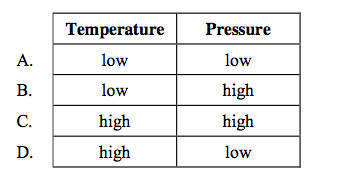For which set of conditions does a fixed mass of an ideal gas have the greatest volume?

D

19

Which of the following contains the greatest number of molecules?
A. 1 g of CH3Cl
B. 1 g of CH2Cl2
C. 1 g of CHCl3
D. 1 g of CCl4

A

20

Which of the following compounds has/have the empirical formula CH2O? I. CH3COOH II. C6H12O6 III. C12H22O11
A. II only
B. III only
C. I and II only
D. II and III only

C

21

Assuming complete reaction, what volume of 0.200 mol dm–3 HCl(aq) is required to neutralize 25.0 cm3 of 0.200 mol dm–3 Ba(OH)2(aq)?
A. 12.5 cm3
B. 25.0 cm3
C. 50.0 cm3
D. 75.0 cm3

C

22

Under what conditions would one mole of methane gas, CH4, occupy the smallest volume?
A. 273 K and 1.01×105 Pa
B. 273 K and 2.02×105 Pa
C. 546 K and 1.01×105 Pa
D. 546 K and 2.02×105 Pa

B

23

The temperature in Kelvin of 2.0 dm3 of an ideal gas is doubled and its pressure is increased by a factor of four. What is the final volume of the gas?
A. 1.0 dm3
B. 2.0 dm3
C. 3.0 dm3
D. 4.0 dm3

A

24

Consider the following equation.
2C4H10(g) + 13O2(g)  8CO2(g) + 10H2O(1)
How many moles of CO2(g) are produced by the complete combustion of 58 g of butane,
C4H10(g)?
A.4
B.8
C.12
D.16

A

25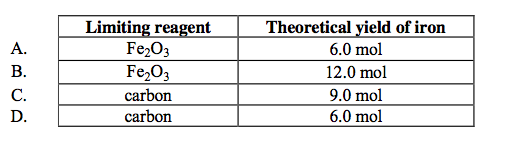6.0 moles of Fe2O3(s) reacts with 9.0 moles of carbon in a blast furnace according to the equation below.
Fe2O3(s) + 3C(s) → 2Fe(s) + 3CO(g)
What is the limiting reagent and hence the theoretical yield of iron?

D

26

What volume of 0.500 mol dm–3 HCl(aq) is required to react completely with 10.0 g of calcium carbonate according to the equation below?
CaCO3(s) + 2HCl(aq) → CaCl2(aq) + H2O(l) + CO2(g)
A. 100 cm3
B. 200 cm3
C. 300 cm3
D.400 cm3

D

27

Which is a correct definition of the term empirical formula?
A. formula showing the numbers of atoms present in a compound
B. formula showing the numbers of elements present in a compound
C. formula showing the actual numbers of atoms of each element in a compound
D. formula showing the simplest ratio of numbers of atoms of each element in a compound

D

28

The reaction of ethanal and oxygen can be represented by the unbalanced equation below. __ CH3CHO + __ O2 → __ CO2 + __ H2O
When the equation is balanced using the smallest possible integers, what is the coefficient for O2?
A. 3
B. 4
C. 5
D. 6

C

29

The equation for the complete combustion of butane is
2C4H10 + 13O2 → 8CO2 + 10H2O
What is the amount (in mol) of carbon dioxide formed by the complete combustion of three moles of butane?
A. 4
B. 8
C. 12
D. 24

C

30

Which solution contains the greatest amount (in mol) of solute?
A. 10.0 cm3 of 0.500 mol dm–3 NaCl
B. 20.0 cm3 of 0.400 mol dm–3 NaCl
C. 30.0 cm3 of 0.300 mol dm–3 NaCl
D. 40.0 cm3 of 0.200 mol dm–3 NaCl

C

31

A fixed mass of an ideal gas has a volume of 800 cm3 under certain conditions. The pressure (in kPa) and temperature (in K) are both doubled. What is the volume of the gas after these changes with other conditions remaining the same?
A. 200 cm3
B. 800 cm3
C. 1600 cm3
D. 3200 cm3

B

32

How many oxygen atoms are present in 0.0500 mol carbon dioxide?
A. 3.01×10^22
B. 6.02×10^22
C. 6.02×10^23
D. 1.20×10^24

B

33

The complete oxidation of propane produces carbon dioxide and water as shown below.
C3H8 + __O2 __CO2 + __H2O
What is the total of the coefficients for the products in the balanced equation for 1 mole of
propane?
A. 6
B. 7
C. 12
D.13

B

34

The relative molecular mass (Mr) of a compound is 60. Which formulas are possible for this compound?
I. CH3CH2CH2NH2 II. CH3CH2CH2OH III.CH3CH(OH)CH3
A. I and II only
B. I and III only
C. II and III only
D. I, II, and III

C

35

35. Which sample has the least number of atoms?
A. 1 mol of H2SO4
B. 1 mol of CH3COOH
C. 2 mol of H2O2
D. 2 mol of NH3

A

36

Avogadro’s constant has the same value as the number of
A. molecules in 1 mol of solid iodine.
B. atoms in 1 mol of chlorine gas.
C. ions in 1 mol of solid potassium bromide.
D. protons in 1 mol of helium gas.

A

37

Which aqueous solution contains the most hydrogen ions?
A. 20 cm3 of 2 mol dm–3 sulfuric acid
B. 10 cm3 of 4 mol dm–3 nitric acid
C. 80 cm3 of 0.5 mol dm–3 hydrochloric acid
D. 40 cm3 of 0.5 mol dm–3 sulfuric acid

A

38

What is the total of all the coefficients in the balanced equation for the reduction of 1 mol of MnO4–?
__ MnO4– +__H+ + __ e– __Mn2+ + __H2O
A. 5
B. 9
C. 17
D. 19

D

39

Which contains the same number of ions as the value of Avogadro’s constant?
A. 0.5 mol NaCl
B. 0.5 mol MgCl2
C. 1.0 mol Na2O
D. 1.0 mol MgO

A

40

A reaction occurring in the extraction of lead from its ore can be represented by this unbalanced equation:
__ PbS + __O2  __ PbO + __ SO2
When the equation is balanced using the smallest possible whole numbers, what is the
coefficient for O2?
A. 1
B. 2
C.3
D.4

C

41

The equation for a reaction occurring in the synthesis of methanol is
CO2 + 3H2  CH3OH + H2O
What is the maximum amount of methanol that can be formed from 2 mol of carbon dioxide and
3 mol of hydrogen?
A. 1 mol
B. 2 mol
C. 3 mol
D. 5 mol

A

42

Which solution contains 0.1 mol of sodium hydroxide?
A. 1 cm3 of 0.1 mol dm–3 NaOH
B. 10 cm3 of 0.1 mol dm–3 NaOH
C. 100 cm3 of 1.0 mol dm–3 NaOH
D. 1000 cm3 of 1.0 mol dm–3 NaOH

C

43

A cylinder of gas is at a pressure of 40 kPa. The volume and temperature (in K) are both doubled. What is the pressure of the gas after these changes?
A. 10 kPa
B. 20 kPa
C. 40 kPa
D. 80 kPa

C

44

Which of the following quantities has units?
A. Relative atomic mass
B. Relative molecular mass
C. Molar mass
D. Mass number

C

45

What is the purpose of the beam of high energy electrons used in a mass spectrometer?
A. To ionize atoms
B. To accelerate ions
C. To deflect ions
D. To detect ions

A

46

The empirical formula of a compound is C2H4O. Which molecular formulas are possible for this compound?
I. CH3COOH II. CH3CH2CH2COOH III. CH3COOCH2CH3
A. I and II only
B. I and III only
C. II and III only
D. I, II and III

C

47

Calcium carbonate decomposes on heating as shown below. CaCO3  CaO + CO2
When 50 g of calcium carbonate are decomposed, 7 g of calcium oxide are formed. What is the percentage yield of calcium oxide?
A. 7%
B. 25%
C. 50%
D. 75%

B

48

Sodium reacts with water as shown below.
__ Na + __ H2O  __ NaOH + __ H2
What is the total of all the coefficients when the equation is balanced using the smallest possible
whole numbers?
A. 3
B. 4
C. 6
D. 7

D

49

What is the total number of ions present in the formula, Al2(SO4)3?
A. 2
B. 3
C. 5
D. 6

C

50

A 4 g sample of sodium hydroxide, NaOH, is dissolved in water and made up to 500 cm3 of aqueous solution. What is the concentration of the resulting solution?
A. 0.1 mol dm–3
B. 0.2 mol dm–3
C. 0.5 mol dm–3
D. 1.0 mol dm–3

B

51

Methane, CH4, burns in oxygen gas to form carbon dioxide and water. How many moles of carbon dioxide will be formed from 8.0 g of methane?
A. 0.25
B. 0.50
C. 1.0
D. 2.0

B

52

What is the empirical formula of a compound containing 50% by mass of element X (Ar = 20)
and 50% by mass of element Y (Ar = 25)?
A. XY
B. X3Y2
C. X4Y5
D. X5Y4

D

53

Assuming complete reaction, what volume of 0.200 mol dm–3 potassium hydroxide solution (KOH(aq)), is required to neutralize 25.0 cm3 of 0.200 mol dm–3 aqueous sulfuric acid,
(H2SO4(aq))?
A. 12.5 cm3
B. 25.0 cm3
C. 50.0 cm3
D. 75.0 cm3

C

54

Consider the following reaction.
N2(g) + 3H2(g) 2NH3(g)
If the reaction is made to go to completion, what volume of ammonia (in dm3) can be prepared
from 25 dm3 of nitrogen and 60 dm3 of hydrogen? All volumes are measured at the same temperature and pressure.
A. 40
B. 50
C. 85
D. 120

A

55

The temperature in Kelvin of 1.0 dm3 of an ideal gas is doubled and its pressure is tripled. What is the final volume of the gas in dm3?
A.1/3
B.2/3
C.3/2
D.1/6

B

56

On complete combustion, a sample of a hydrocarbon compound produces 1.5 mol of carbon
dioxide and 2.0 mol of water. What is the molecular formula of this hydrocarbon?
A. C2H2
B. C2H4
C. C3H4
D. C3H8

D

57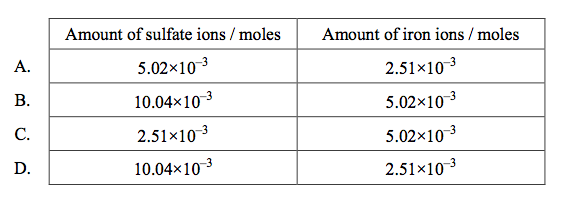When excess BaCl2(aq) was added to a sample of Fe(NH4)2(SO4)2(aq) to determine the amount in moles of sulfate present, 5.02×10–3 mol of BaSO4 was obtained. How many moles of sulfate
ions and iron ions were in the sample of Fe(NH4)2(SO4)2?

A

58

What volume of 0.500 mol dm–3 sulfuric acid solution is required to react completely with 10.0 g of calcium carbonate according to the equation below?
￼￼￼CaCO3(s) + H2SO4(aq)  CaSO4(aq) + H2O(l) + CO2(g)
A. 100 cm3
B. 200 cm3
C. 300 cm3
D. 400 cm3

B

59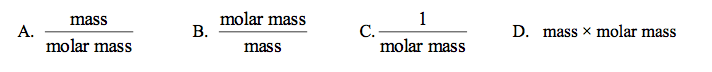Which expression gives the amount (in mol) of a substance, if the mass is given in grams?

A

60

What is the total number of atoms in 0.20 mol of propanone, CH3COCH3?
A. 1.2×10^22
B. 6.0×10^23
C. 1.2×10^24
D. 6.0×10^24

C

61

When the equation below is balanced for 1 mol of C3H4, what is the coefficient for O2?
C3C4 + _O2 -> _CO2 + _H2O
A.2
B.3
C.4
D.5

C

62

Ethyne, C2H2, reacts with oxygen according to the equation below. What volume of oxygen (in dm3) reacts with 0.40 dm3 of C2H2?
2C2H2(g) + 5O2(g)  4CO2(g) + 2H2O(g)
A. 0.40
B. 0.80
C. 1.0
D. 2.0

C

63

Ethyne, C2H2, reacts with oxygen according to the equation below. What volume of oxygen (in dm3) reacts with 0.40 dm3 of C2H2?
2C2H2(g) + 5O2(g)  4CO2(g) + 2H2O(g)
A. 0.40
B. 0.80
C. 1.0
D. 2.0

C

64

What is the coefficient for H+ when the redox equation below is balanced?
_Ag(s) + _NO3–(aq) +_H+(aq) -> _Ag+(aq) + _NO(g) + _H2O(l)
A.1
B.2
C.3
D.4

D

65

How many hydrogen atoms are in one mole of ethanol, C2H5OH?
A. 1.00×10^23
B. 3.61×10^24
C. 5.00
D. 6.00

B

66

What is the coefficient for H2SO4(aq) when the following equation is balanced, using the smallest possible integers?
__Mg3N2(s) + __H2SO4(aq) → __MgSO4(aq) + __(NH4)2SO4(aq)
A.1
B.3
C.4
D.7

C

67

Air bags in cars inflate when sodium azide decomposes to form sodium and nitrogen: 2NaN3(s) → 2Na(s) + 3N2(g)
Calculate the amount, in moles, of nitrogen gas produced by the decomposition of 2.52 mol of NaN3(s).
￼￼A. 1.68
B. 2.52
C. 3.78
D. 7.56

C

68

What volume, in cm3, of 0.200 mol dm–3 HCl(aq) is required to neutralize 25.0 cm3 of 0.200 mol dm–3 Ba(OH)2(aq)?
A. 12.5
B. 25.0
C. 50.0
D. 75.0

C